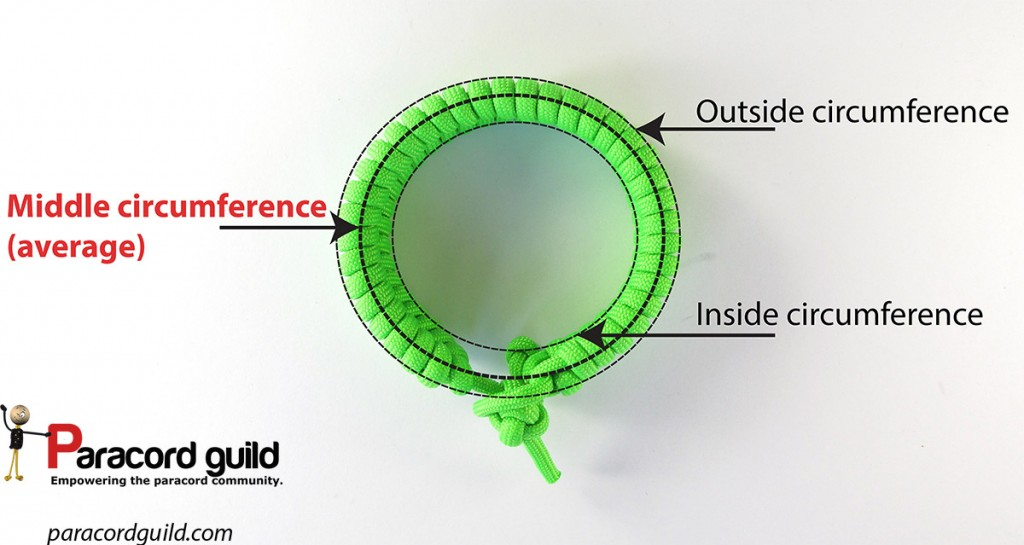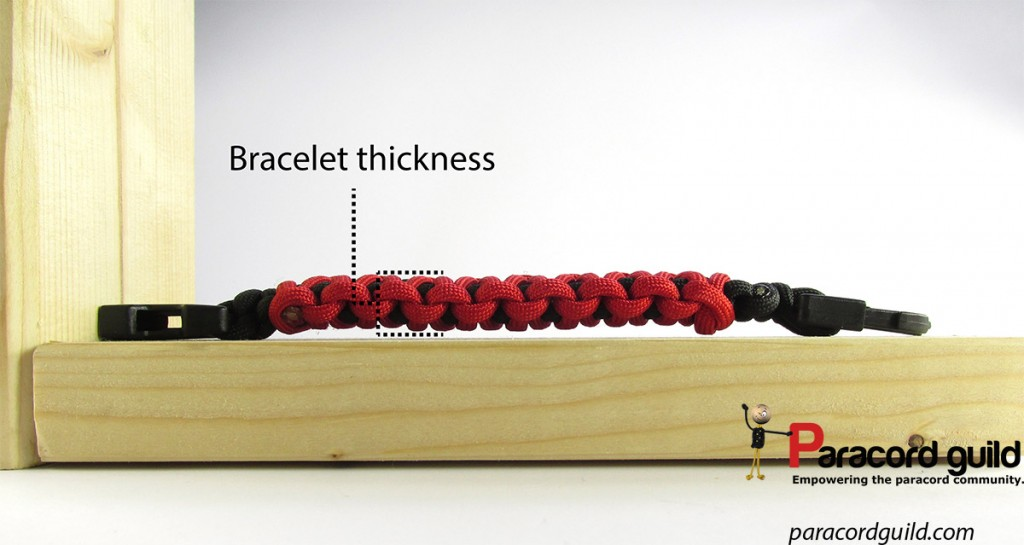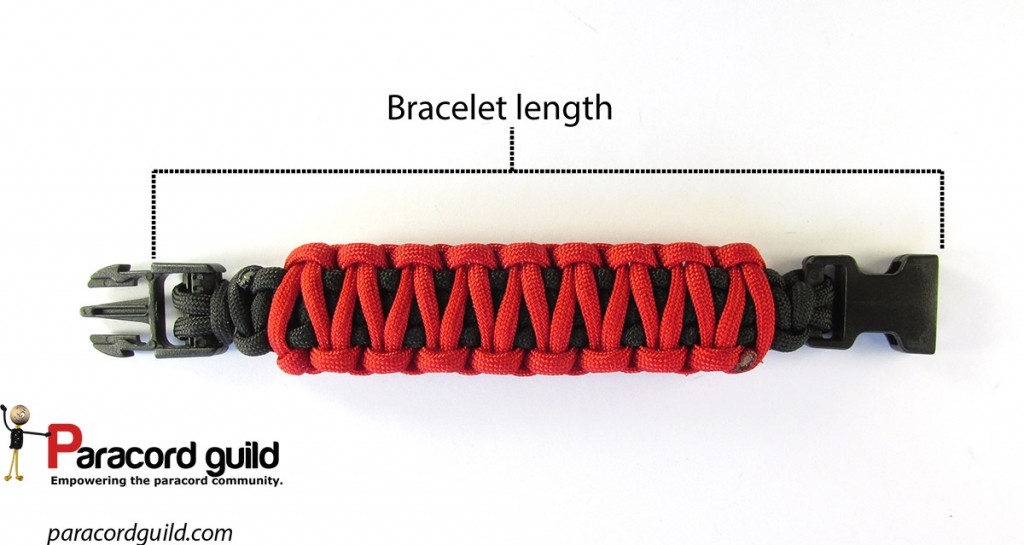Don't Miss

# Bracelet sizing- how long should a bracelet be to fit?

Making a bracelet for yourself is easy, you can adjust it as you are tying it. The problem of sizing bracelets becomes apparent when you are making bracelets for other people. Because the wrist sizes vary, you will need a method for calculating what bracelet length you need for a specific wrist size. The whole point of doing this is to avoid getting your bracelets returned due to a bad fit.

### The problem

A 6 inch bracelet will not fit a wrist that is 6 inches in circumference. It is too small.

The issue is the thickness of the bracelet. You can easily test this by taking a piece of paper and wrap it around your wrist. Mark your wrist size on the paper.

Your wrist size and the length of the paper strip will be about the same. This is due to the fact that the paper strip has almost no thickness.

A bracelet the same length as the paper strip will not fit you at all.Due to the thickness of the bracelet, the inner circumference of a bracelet is smaller than that of the assumed, middle one.

What we are looking for when making a bracelet is the length of the bracelet for a specific wrist circumference. We measure this by laying our bracelet out and measuring all the parts that add to the length of it (basically the weave and the buckle).

### Thickness

So, the problem is in the thickness of the bracelet. When we have that, we can then use a simple equation to get the bracelet length we need.

So how do you measure the thickness of your bracelet? Simply make a small length, then measure it. Yes, it is that simple!### Solving the required bracelet lengthWhen determining your bracelet length we are going to use a simple equation found below. Another piece of information, that should also be included in the bracelet length calculation is the “fit”.

Basically, we know two fits:

• close fit, also called a snug fit is a fit where the bracelet is not able to move much on the wrist. This is achieved by making a bracelet the exact length as calculated below
• loose fit, where the bracelet is larger than the wrist. This makes it less tight. To achieve this you add anywhere from 1/16 of an inch to 1/4 inches to the length of the “snug fit” to get a loose fitting bracelet.

To determine the bracelet size you need use the following equation:

The length of the bracelet= Wrist size+(3.14159*thickness of the bracelet)

### Breaking it down and example

So, we got a wrist measurement from a client. We also know the thickness of the bracelet, since we measured it. We simply insert that information into the equation and off we go!

#### An example

We got a wrist measurement of 6 inches. We measured the bracelet thickness of the design we are making, and it was at 0.375 inches. We also know that we need to make a snug bracelet, meaning we will not add to the total length to make it a loose fit.

With that information, we crunch the numbers:

The length of the bracelet= 6+ (3.14159*0.375)

The length of the bracelet=7.18 inches

So for a 6 inch wrist of the stated thickness, we need to make a 7.18 inch bracelet. Note that the actual weave will be less than that, since the total length includes a buckle!

### In conclusion

The simple equation above will help you make bracelets of proper length. Getting bracelets returned is not in the interest of you, or your customers. Using this simple method means you will save funds and time.I am a defense science graduate. I like to create beautiful things out of paracord.

1.Hi please could you explain sam scafferi’s way of measuring paracord bracelet’s? 6+ (3.14159*0.375) how do you get 7.18″? How are you calculating the bracketed bit cheers

•Hey!

Well, Pi=3,14 and multiplied by 0,375 is about 1,18.

Mark

2.thank you for this clear and practical explanation!

3.This’ll come in handy!

4.Brilliant. I do not make Paracord bracelets, but this is the most helpful thing I’ve found that gives a formula for calculating bracelet length when using any large beads or stringing material.

5.Thank you so much… That “Pi ” comes in handy, once again ! Just plain logical, as well! Much appreciated.

6.Thank you, Mark!!!!! I just wish I’d found this information BEFORE a friend and I launched into a project that has totaled over 300 paracord bracelets to this point in time!!!

Our biggest challenge is – and has been – how to get bracelets to finish to the size we want. We’re close – but sometimes “close” isn’t good enough.

In all of my searching, this is the ONLY information I have found on paracord bracelet sizing. (And to think I ordered a jig today – hoping that would help!)

7.Hello! I’m Maria from Hungary. I like the website very much. I would like to ask for help. How do I calculate the amount of material needed? I would like to see how much less the loss at the time of making. Thank you for your reply.

•Hey Maria!

Honestly It depends on the bracelet pattern, length, how tight you tie it and so on. Usually I will tie a few test lengths to see how much cord I use per inch and then extrapolate from that for a specific length.

Mark

8.Isn’t Pi*thickness measuring the addition to radius? I’ve used 2Pi*thickness to get a proper length for diameter.

9.Normally, circumference (here the length of the bracelet) is calculated with 2 x Pi x r.
That does not take thickness of used material into account, as explained by the author.

In this case you do not need the outer circumference of a bracelet with a given thickness, but the average between it and the wrist circumference as you are trying to figure out the length of the core strands of the bracelet. That’s halfway the thickness of the bracelet.

So the added length is actually half of the difference you would calculate with wristsize vs outer bracelet circumference.
That means (2 x Pi x r) divided by 2. That’s the same as Pi x r.

Example in numbers:

Let’s say your wristsize (circumference) is 20cm.
This would be the inner size of your bracelet.
20 = 2 x Pi x r
20 = 2 x Pi x 3,183

Assume a bracelet thickness of 1 cm, adding 1 cm to the radius of your wristsize, making r now 4,183.